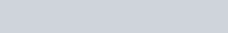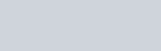In a rectangle, if the length is increased by 3 meters and breadth is decreased by 4 meters,
Question:

In a rectangle, if the length is increased by 3 meters and breadth is decreased by 4 meters, the area of the rectangle is reduced by 67 square meters. If length is reduced by 1 meter and breadth is increased by 4 meters, the area is increased by 89 Sq. meters. Find the dimensions of the rectangle.

Solution:

Let the length and breadth of the rectangle be $x$ and $y$ units respectively

Then, area of rectangle $=x y$ square units

If the length is increased by 3 meters and breath is reduced each by 4 square meters the area is reduced by 67 square units

Therefore,

$x y-67=(x+3)(y-4)$

$x y-67=x y+3 y-4 x-12$$4 x-3 y-67+12=0$

$4 x-3 y-55=0 \cdots(i)$

Then the length is reduced by 1 meter and breadth is increased by 4 meter then the area is increased by 89 square units

Therefore, $0=4 x-y-93 \cdots(i i)$

Thus, we get the following system of linear equation

$4 x-3 y-55=0$

$4 x-y-93=0$

By using cross multiplication we have

$\frac{x}{(-3 \times-93)-(-1 \times-55)}=\frac{-y}{(4 \times-93)-(4 \times-55)}=\frac{1}{(4 \times-1)-(4 \times-3)}$

$\frac{x}{279-55}=\frac{-y}{-372+220}=\frac{1}{-4+12}$$x=\frac{224}{8}$

$x=28$

and

$y=\frac{152}{8}$

$y=19$

Hence, the length of rectangle is 28 meter,

The breath of rectangle is 19 meter.